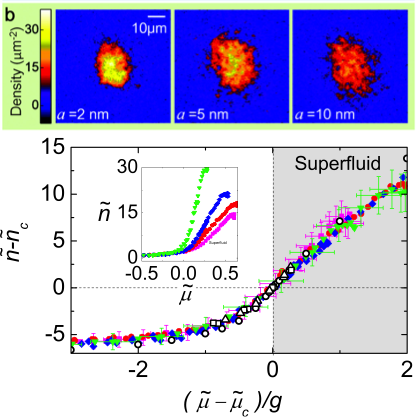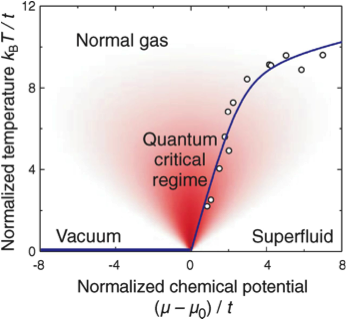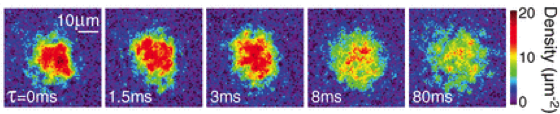### Hung Lab@PurdueUltracold quantum gas and quantum optics

Quantum Gas and Quantum Optics » Research » Quantum Gas Research

### Prior results on quantum gases

One important step toward quantum simulation using atomic quantum gases was the ability to extract local information of trapped samples beyond conventional time-of-flight bulk measurements. My research provided a solution by developing a high-resolution, in situ imaging scheme for atomic samples in a two-dimensional (2D) configuration. We obtained one of the first in situ observations, in a single snapshot, of the ‘wedding cake structure’ of trapped atomic samples undergoing a superfluid-to-Mott insulator quantum phase transition . Our technique enabled detail studies of equilibrium and non-equilibrium thermodynamics in strongly correlated systems [2, 3]. We also developed a new method to extract collective and statistical behavior of many-body phases by analyzing in situ density fluctuations and correlations . With this new set of tools, we further observed the following exciting phenomena:

Observation of scale invariance and universality in 2D Bose gases

 We studied universal scaling symmetries of weakly interacting 2D Bose gases near the Berezinsky–Kosterlitz–Thouless (BKT) transition. By using atomic samples prepared at different temperatures T, chemical potentials μ, and interaction strengths, we tested universal scaling behavior based on density observables such as the equations of state and the quasi-condensate density derived from density fluctuation measurements. Our results verified calculations based on a classical φ4-field theory, a paradigm model describing superfluid critical behavior in both two and three spatial dimensions. Transition from simple to renormalized universal scaling behavior was measured in  using strongly interacting 2D gases. Figure 1 Scale invariance and universality in 2D Bose gases. Upper panel shows density images of trapped atoms at different interaction strength (controlled by atomic scattering length a). Lower panel shows the measured equations of state (filled symbols) and their scaling to a universal curve near the BKT critical point. Open symbols mark theory calculations. Figure is adapted from C.-L. Hung, X. Zhang, N. Gemelke and C. Chin, Nature 470, 236 (2011) .Observation of quantum criticality with cold atoms in optical lattices

 The test of critical universality was carried into the realm of quantum criticality . By loading an atomic superfluid into a 2D square optical lattice, we studied critical scaling behavior near a superfluid-to-Mott insulator (zero occupancy vacuum state) quantum phase transition (QPT). Despite the apparent broken translational symmetry due to the lattice potential, at ultra-low temperatures below the lattice ground-band energy width, we observed that the equations of state scale universally with temperature, as it is the sole dominant energy scale near the quantum critical point. Figure 2 Phase diagram of a vacuum-to-superfluid quantum phase transition in 2D. At zero temperature, vacuum (horizontal line) to superfluid QPT occurs at a critical chemical potential μ0. Close to the critical point, quantum criticality prevails (shaded area), and the superfluid critical temperature Tc (open circles) vanishes as Tc ~ (μ − μ0)zν, where the critical exponents z and ν are determined experimentally. Figure is from X. Zhang, C.-L. Hung, S.-K. Tung and C. Chin, Science 335, 1070 (2012) .Cold atom cosmology: observation of Sakharov acoustic oscillations in a quenched atomic superfluid

 Sakharov oscillations, conventionally discussed in the context of early universe evolution and the anisotropy of cosmic microwave background (CMB) radiation, are a consequence of the interference of acoustic waves synchronously generated in an ideal fluid. In atomic superfluid, we induced and observed Sakharov oscillations by quenching the atomic interactions and by monitoring the subsequent time evolution in the density noise power spectrum. The interaction quench-down (up) simulates an inflationary (deflationary) process. The fluctuating superfluid density corresponds to the amplified primordial quantum fluctuations that seed the temperature anisotropy in CMB and the large-scale structure of the universe. This work promises new ways of using atomic superfluids to study analogue phenomena predicted in cosmology and gravitational physics.Figure is from C.-L. Hung, V. Gurarie and C. Chin , Science  341, 1213 (2013) .Figure 3 Single-shot density images of quenched superfluid samples recorded after various hold time t. Sakharov acoustic oscillations are observed in both spatial and time domain.

References

 N. Gemelke, X. Zhang, C.-L. Hung and C. Chin, In situ observation of incompressible Mott-insulating domains in ultracold atomic gases. Nature 460, 995 (2009).

 C.-L. Hung, X. Zhang, N. Gemelke and C. Chin, Slow mass transport and statistical evolution of an atomic gas across the superfluid–Mott-insulator transition. Phys. Rev. Lett. 104, 160403 (2010).

 L.-C. Ha, C.-L. Hung, X. Zhang, U. Eismann, S.-K. Tung and C. Chin, Strongly interacting two-dimensional Bose gases. Phys. Rev. Lett. 110, 145302 (2013).

 C.-L. Hung, X. Zhang, L.-C. Ha, S.-K. Tung, N. Gemelke and C. Chin, Extracting density–density correlations from in situ images of atomic quantum gases. New J. Phys. 13, 075019 (2011).

 C.-L. Hung, X. Zhang, N. Gemelke and C. Chin, Observation of scale invariance and universality in two-dimensional Bose gases. Nature 470, 239 (2011).

 S. Sachdev, Quantum Phase Transitions (Cambridge Univ. Press., Cambridge,  2011).

 X. Zhang, C.-L. Hung, S.-K. Tung and C. Chin, Observation of quantum criticality with ultracold atoms in optical lattices. Science 335, 1070 (2012).

 C.-L. Hung, V. Gurarie and C. Chin, From cosmology to cold atoms: observation of Sakharov oscillations in a quaneched atomic superfluid. Science 341, 1213 (2013).